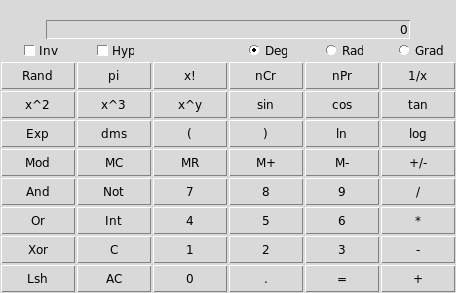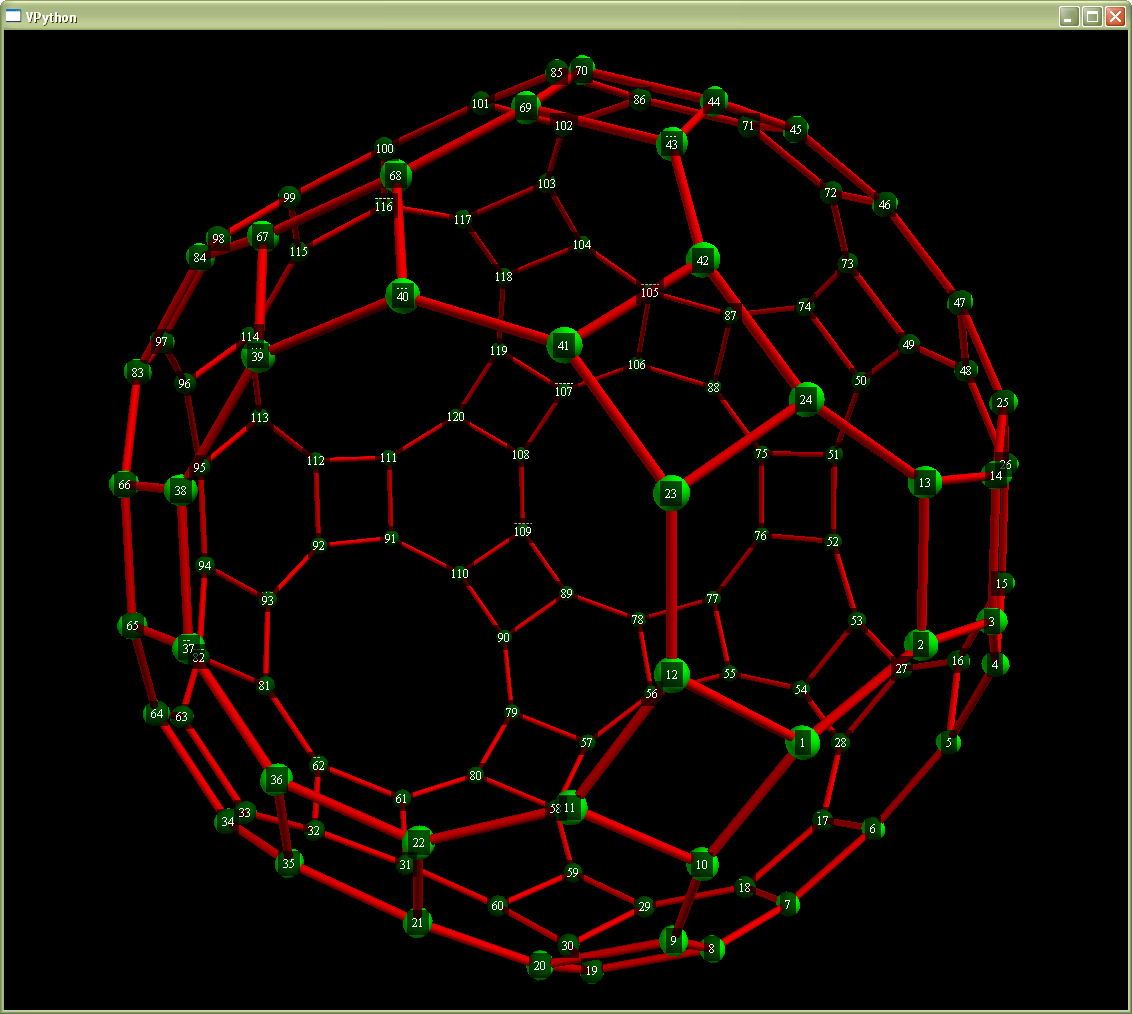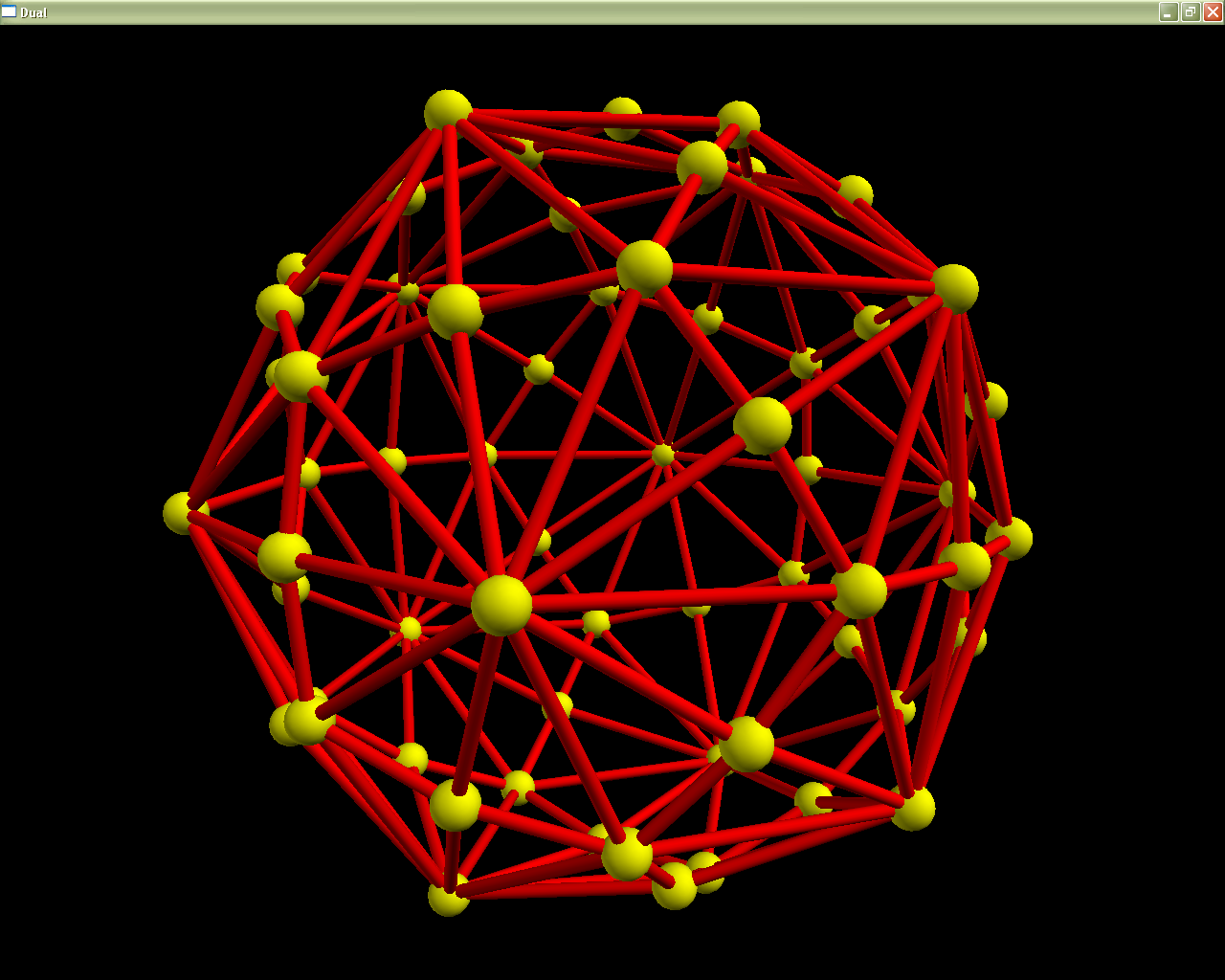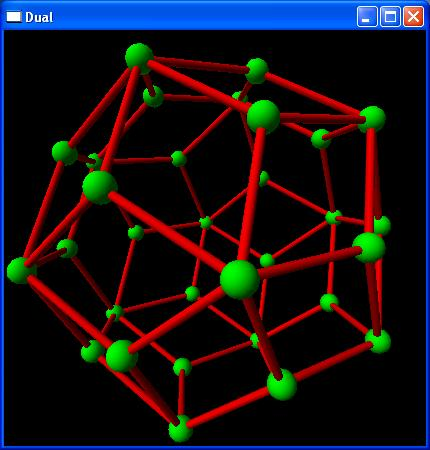# Math Activities

## Universal Algebra

Universal algebra is a generalized study of algebraic structures. The following PDFs show the significance of subalgebras, products, homomorphisms and much more. Exercises are at the end of every section.
• Section 1 (Definition and Examples of Ω-algebras). Introduces the signature and Ω-algebra, after a page-long hook. Then, shows extension signatures and the direct product.
• Section 2 (Subalgebras and Products). Defines a subalgebra and shows their lattice structure, then defines direct products in general.
• Section 3 (Homomorphisms and Isomorphisms). Introduces homomorphisms and isomorphisms, proves fundamental things about them, and shows the surjectification theorem.
• Section 4 (Congruence Relations). Introduces congruence relations and shows their lattice structure.
• Section 5 (Quotient Algebras and Homomorphisms). Shows how to factor a congruence relation to obtain a quotient algebra. Then, shows the injectification theorem and proves the isomorphism theorems.
• Section 6 (Subdirect Products). Studies subdirect products, mainly for Section 10. Also covers subdirect irreducibility.
• Section 7 (The Ultraproduct). Studies ultraproducts and shows that they preserve logical axioms better than direct products. This section is completely elective.
• Section 8 (Ω-expressions and Free Ω-algebras). Introduces the Ω-expression as a mathematical object, and the universal mapping property for a free Ω-algebra.
• Section 9 (Varieties and Coproducts). Defines what a variety is, shows closure under homomorphic images, subalgebras and products, and shows the existence of coproducts.
• Section 10 (Birkhoff's Theorem). Shows that a class of Ω-algebras containing T(Ω) and closed under homomorphic images, subalgebras and products is a variety. Exercise 3 is dedicated to Yoneda's Lemma in category theory.
• Section 11 (Takeoffs and Universals). Studies special maps posessed by varieties, as opposed to the algebras in them; along with a recipe for finding universals.

## Category Theory

Category theory is a mathematical study of objects with special kinds of structure, and it is secretly everywhere in mathematics. The following PDFs go over limits, Yoneda's lemma, monads, algebraic categories and many other things.
• Section 1 (Definition and Examples of Categories). Introduces categories, though it uses universal algebra for motivation.
• Section 2 (Monic and Epic Morphisms). Defines monic and epic morphisms, and proves some things about them.
• Section 3 (Functors and Natural Transformations). Introduces functors and natural transformations, and uses them to construct more categories.
• Section 4 (Products and Coproducts). Shows the definition of a product/coproduct in an arbitrary category.
• Section 5 (Universals). Covers the universal from an object to a functor, along with its dual.
• Section 6 (Limits and Colimits). This section introduces limits and colimits in general and proves they always exist in a variety.
• Section 7 (Hom Functors, Yoneda's Lemma and Representability). Introduces hom functors hom(A,—) and covers Yoneda's Lemma and representable functors.
• Section 8 (Adjunctions). Introduces adjoint functors, and shows many ways they could be thought of.
• Section 9 (Concrete Categories). This section concerns concrete categories, and goes in depth what wouldn't be immediately applicable in an arbitrary category.
• Section 10 (Algebraic Categories). This section shows that a concrete category satisfying certain conditions must be a variety.
• Section 11 (Monads). Introduces monads, with a lot of informal describing. Also shows how a monad can be gotten from an adjunction and vice versa, and introduces the Eilenberg-Moore category.
• Section 12 (Monads on Set). This section intends to classify the monads on the category Set of sets.

## Calculator

calculator.py (updated 12/4/2010) is a scientific calculator I've written. It is a Calculator with Trail, meaning that intermediate results are printed to a separate window as you go along. It uses the order of operations: 2 + 3 * 3 = gives you 2 + (3 × 3) = 11, not (2 + 3) × 3 = 15. It supports 26 levels of parentheses.

It is one of these tools used for solving and checking. I started this in Java, and then tried Visual Basic, but found a much easier way to implement this here in Python!

Here is a screenshot:To use the calculator:

• Download calculator.py to your computer. Its real name will be calculator.py.txt, because the server doesn't want to store executable Python scripts.
• Rename the file by removing the .txt from the name. Its name should be calculator.py.
• If you have an icon for calculator.py on your desktop, double-click on it. Or bring up a terminal (DOS window, Linux window, etc.) and type calculator.py. On Linux, make sure the file is executable (chmod 755 calculator.py). If all else fails, open a terminal and type python calculator.py.

## Slide Rule

A slide rule lets you do multiplication, division, powers, roots, and other functions, just by sliding parts of the rule around and reading numbers off the scales. It is all based on logarithms, since multiplying numbers is the same as adding their logarithms.

To use my slide rule, download sliderule.py and follow the same instructions as for the calculator above.

To move the slide in my slide rule, hold down the left mouse button and drag the "slide", the middle section with the C and CI, from side to side. To move the red hairline, hold down the right button and drag.

Here are some examples.

• To multiply 2×3, drag the C scale with the left mouse button until the 1 on the left side of the C is over the 2 on the D scale. With the right mouse button, drag the red hairline till it is over the 3 on the C. You will see that the red line is over the 6 on the D scale. Yes, 2×3 = 6.
• With the red line on the 6 on the D, notice that the red line is over 36 on the A scale. This shows 62 = 36. Conversely, √36 is 6.
• Similarly, with the red line on the 6 on the D, notice that the red line is over 216 on the K scale. This shows 63 = 216. Conversely, 3√216 = 6.
Please see Eric's Slide Rule Site for information about the other scales.

We wrote this in 2010. Dad wrote the moving parts of the slide rule and the mouse motions, and he added the C scale. I added the other scales.

## Polyhedra

I really enjoyed polyhedra when I was in 4th and 5th grade. I still love them now. Here are some of the polyhedra I played with back then. Dad wrote a program in Visual Python to make pictures like these. I worked out the details of how to join the vertices and edges together. Here is the code.

### The Rhombicosidodecahedron### The Disdyakis Triacontahedron

This is the dual of the rhombicosidodecahedron. Every vertex in the first picture matches a face of this one, and every face in the first picture matches a vertex of this one.### A Die with Thirty Sides

My sister and her friends use this kind of die to play Dungeons and Dragons.